# Python | Pandas TimedeltaIndex.union()

• Last Updated : 29 Sep, 2021

Python is a great language for doing data analysis, primarily because of the fantastic ecosystem of data-centric python packages. Pandas is one of those packages and makes importing and analyzing data much easier.

Pandas TimedeltaIndex.union() function is a specialized union for TimedeltaIndex objects. It combines overlapping ranges with the same DateOffset, will be much faster than Index.union

Syntax : TimedeltaIndex.union(other)
Parameters :
other : TimedeltaIndex or array-like
Return : Index or TimedeltaIndex

Example #1: Use TimedeltaIndex.union() function to find the set union of two TimedeltaIndex objects.

## Python3

 `# importing pandas as pd``import` `pandas as pd` `# Create the first TimedeltaIndex object``tidx1 ``=` `pd.TimedeltaIndex(start ``=``'11 days 22:11:12.001124'``,``                         ``periods ``=` `5``, freq ``=``'T'``, name ``=``'New_object'``)` `# Create the second TimedeltaIndex object``tidx2 ``=` `pd.TimedeltaIndex(start ``=``'11 days 22:14:12.001124'``,``                         ``periods ``=` `5``, freq ``=``'T'``, name ``=``'New_object'``)` `# Print the first TimedeltaIndex object``print``(tidx1)` `# Print the second TimedeltaIndex object``print``(tidx2)`

Output :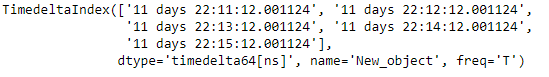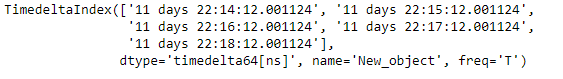Now we will use the TimedeltaIndex.union() function to find the set union of the two objects

## Python3

 `# find the union``tidx1.union(tidx2)`

Output :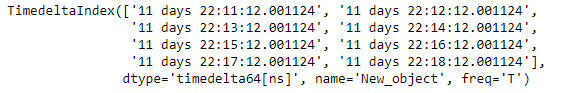As we can see in the output, the TimedeltaIndex.union() function has returned an object which contains the union of both tidx1 and tidx2.

Example #2: Use TimedeltaIndex.union() function to find the set union of two TimedeltaIndex objects.

## Python3

 `# importing pandas as pd``import` `pandas as pd` `# Create the first TimedeltaIndex object``tidx1 ``=` `pd.TimedeltaIndex(start ``=``'1 days 02:00:12.001124'``,``                         ``periods ``=` `5``, freq ``=``'D'``, name ``=``'Koala'``)` `# Create the second TimedeltaIndex object``tidx2 ``=` `pd.TimedeltaIndex(start ``=``'3 days 02:00:12.001124'``,``                         ``periods ``=` `5``, freq ``=``'D'``, name ``=``'Koala'``)` `# Print the first TimedeltaIndex object``print``(tidx1)` `# Print the second TimedeltaIndex object``print``(tidx2)`

Output :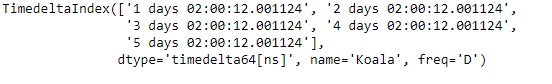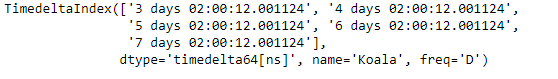Now we will use the TimedeltaIndex.union() function to find the set union of the two objects

## Python3

 `# find the union``tidx1.union(tidx2)`

Output :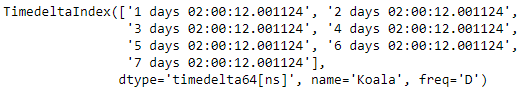As we can see in the output, the TimedeltaIndex.union() function has returned an object which contains the union of both tidx1 and tidx2.

My Personal Notes arrow_drop_up